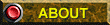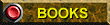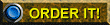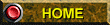Ken's Electronics, (Wholesale & Retail Electronic Parts) Kalamazoo, Michigan

To SEARCH (on this page): Press Ctrl + F keys (at same time), type item wanted in "Find" box.

This webpage is updated often to show our current inventory!

What if your vintage crystal police scanner is a different brand than Regency, Radio Shack Realistic, or Electra Bearcat? Look at the markings on existing/working crystals then: Click here for CRYSTAL COMPATIBILITY information.

If you have an ancient vintage binary-programmable scanner such as an Electra Bearcat 101, Radio Shack Realistic COMP-100, Regency "Whammo" ACT-W10, SBE "OPTI-SCAN" SBE12SM, or Tennelec MS-1 or MS-2? You may want to order a copy of the appropriate manual. Click here for PROGRAMMING MANUALS.

 Specify RADIO make & model so we can send the right crystals for your unit Police Regency RG 10.7MHz IF (Regency & Realistic) High & Low VHF crystals NOS numbers below \$10.00 Police Bearcat BC 10.8MHz IF (Electra Bearcat III & IV etc) High & Low VHF crystals NOS numbers below \$10.00 Aircraft, Amateur (Ham), Marine, Weather, & UHF, in-stock NOS numbers listed *\$15.00 Special Order crystals (frequencies not listed) Ask about place to order

Regency & Radio Shack Realistic (10.7MHz IF RG-type) scanner crystals:RG Regency Radio Shack Realistic Low Band NOS crystals \$10 each except as shown
RG-31.340
RG-31.960
RG-33.080&RCB
RG-33.430
RG-33.720
RG-33.760
RG-33.780=V
RG-33.900
RG-33.980
RG-34.420
RG-35.080
RG-35.100
RG-35.420
RG-35.700-SR-E4
RG-35.720
RG-35.880
RG-35.980
RG-37.100
RG-37.120=
RG-37.180
RG-37.200
RG-37.220
RG-37.260
RG-37.340=XV
RG-37.400
RG-37.420
RG-37.560
RG-37.620
RG-37.680
RG-37.720=V
RG-37.800=WTV
RG-37.900
RG-37.940
RG-37.980=V
RG-38.580=XX
RG-38.880
RG-39.020
RG-39.060
RG-39.240
RG-39.280
RG-39.300
RG-39.320=X-PB
RG-39.340
RG-39.360
RG-39.380=PB
RG-39.420=PB
RG-39.460=V&PB
RG-39.500=XXXV-PB
RG-39.520=PB
RG-39.560
RG-39.580=V
RG-39.590=RCB
RG-39.660=XX-PB
RG-39.750
RG-39.760
RG-39.820=XX=
RG-39.860
RG-39.920
RG-39.940
RG-40.000
RG-41.120=V
RG-41.710
RG-42.100
RG-42.120
RG-42.160=X=
RG-42.220=V-PB JK-1 RG-42.220-JE2 MCS-1=JE1 MRL-1=JE5 MRL-1=JE1 RCD-1=JE2
RG-42.260=XXX=
RG-42.380=XXX= JK-1 RG-42.380-JE1 MCS-1=JE1 MRL-1=JE4 MRL-1=JE2 RCD-1=JE3
RG-42.420=XL=
RG-42.450
RG-42.470
RG-42.480=L= &RCB
RG-42.500=XXX=
RG-42.520=XV-PB
RG-42.550
RG-42.560=XXX= &RCB
RG-42.580=XXX =
RG-42.620=V-PB
RG-42.640
RG-42.660=X-PB
RG-42.680
RG-42.740=XXXX
RG-42.760
RG-42.800
RG-42.960
RG-43.000
RG-43.480
RG-43.560
RG-44.020=WTV
RG-44.540
RG-44.600
RG-44.620
RG-44.640=V
RG-44.720
RG-44.740=WTV
RG-44.860
RG-44.940=V
RG-45.020
RG-45.140=
RG-45.160=XV=
RG-45.180
RG-45.260=V=
RG-45.440
RG-45.460=
RG-45.540
RG-45.560
RG-45.580
RG-45.660
RG-45.680
RG-45.700
RG-45.780
RG-45.820
RG-45.860
RG-45.940
RG-46.040&RO
RG-46.18=RO
RG-46.200=V
RG-47.280=X=
RG-47.360=XXV=
RG-47.380
RG-47.500=X=
RG-46.540
RG-47.580=V=
RG-47.600=V=
RG-47.740=XXXX=
RG-47.780=Regency AIR Aircraft Band NOS crystals: (*\$15)
*116.25
AIR*118.20
AIR*118.300
AIR*118.400
AIR*118.50
AIR*118.950

AIR*119.40
AIR*121.200
AIR*121.700
AIR*121.900
AIR*123.000
AIR*124.250
AIR*126.100
AIR*127.25
AIR*127.550
AIR*128.500
AIR*134.140
AIR*134.46
AIR*135.900RG Regency TMR, Radio Shack Realistic Ham Radio 2-Meter NOS crystals: (*\$15)
RG-*144.830-bx
RG-*145.170
RG-*145.595-bx
RG-*146.040
RG-*146.130
RG-*146.150=PR
RG-*146.160=V
RG-*146.190
RG-*146.220=V
RG-*146.280
RG-*146.310=V
RG-*146.325-bx
RG-*146.340
RG-*146.385-bx
RG-*146.400
RG-*146.430
RG-*146.460-XXV-PB
RG-*146.520= MRH-1 RG-146.520-JE6
RG-*146.610=
RG-*146.640=
RG-*146.670=V
RG-*146.675-bx
RG-*146.730=

RG-*146.790=X
RG-*146.850=V
RG-*146.875-RS RG-*146.880=
RG-*146.940=X
RG-*146.985-bx
RG-*147.000=V

RG-*147.270-XV=
RG-*147.420=
RG-*147.480-bx
RG-*147.555-bx
RG-*147.600-bx
RG-*147.750-bx
RG-*147.870=
RG-*147.990-bxRG Regency TMR Radio Shack Realistic High Band NOS crystals \$10.00 each except as shown
RG-148.100-PR
RG-148.150-SR-E4
RG-148.290-SR-E4
RG-148.410
RG-149.450-SR-E4
RG-149.470
RG-151.030-PR
RG-151.085
RG-151.115=X-PB
RG-151.150
RG-151.460
RG-151.655-WTV
RG-151.695
RG-151.715
RG-151.745-PB&SR-E4

RG-151.775
RG-151.805-PR
RG-151.835-PR &RS
RG-151.895-PR&SR-E4

RG-151.925-PR
RG-151.955-WTV &PR
RG-152.030=V
RG-152.090=XXV &PR
RG-152.150
RG-152.210-PR
MRH-1 RG-152.240-JE1
RG-152.250-SR-E4
RG-152.270-PR
RG-152.300-PR
RG-152.360
RG-152.390-PR
RG-152.420=XV &PR
RG-152.480-PR
RG-152.510=&SR-E4

RG-152.540-SR-E4
RG-152.570=X &RCB &PR &SR-E4

RG-152.600-SR-E4
RG-152.625
RG-152.630
RG-152.660-SR-E4
RG-152.690-WTV&SR-E4

RG-152.720-SR-E4
RG-152.735
RG-152.745
RG-152.750=V&SR-E4
RG-152.780=&SR-E4
RG-152.810=XXV&SR-E4
RG-152.900-SR-E4
RG-152.945-PR
RG-152.990
RG-153.030
RG-153.035-PB
RG-153.065-PR
RG-153.130 &PR&SR-E4
RG-153.200-PR
RG-153.350-WTV
RG-153.365-PR
RG-153.590-bag
RG-153.600-PR
RG-153.740-PR
RG-153.765
RG-153.785=X (Kazoo area fire)
RG-153.810-PR
RG-153.815
RG-153.835
RG-153.840-SR-E4
RG-153.860-PR
RG-153.880=
RG-153.890= &RCB
RG-153.905=
RG-153.920=XX
RG-153.930-PR
RG-153.955-RS
RG-153.980=V
RG-153.995-PR
RG-154.000-SR-E4
RG-154.025-SR-E4
RG-154.055-RS
RG-154.070
RG-154.080=V-PB&SR-E4

RG-154.100=&SR-E4

RG-154.115 MCS-1 RG-154.115-JE1 MCS-1=JE2 MRH-1=JE1
RG-154.155-RS
RG-154.160-V
RG-154.180-SR-E4
RG-154.230-SR-E4
RG-154.250-X=
RG-154.260=
RG-154.300 -V

RG-154.320= &RS
RG-154.330-RS
RG-154.365-SR-E4
RG-154.385-RS
RG-154.390
 RG-154.400
RG-154.405
 RG-154.430
RG-154.440-RS
RG-154.450-SR-E4
RG-154.460
RG-154.475=&SR-E4
RG-154.490-RS=\$15
RG-154.515=V
RG-154.540-V
RG-154.545
RG-154.575
RG-154.600=&-SR-E4

RG-154.625-SR-E4
RG-154.630-RS
RG-154.640-RS
 RG-154.650
 RG-154.655
RG-154.680-SR-E4 & KO-Misc
RG-154.695-SR-E4
 RG-154.710
RG-154.730=V
RG-154.735-RS
 RG-154.740
RG-154.745
RG-154.750-RS
 RG-154.755
 RG-154.760-RS
 RG-154.770
RG-154.775= &RS
RG-154.790
RG-154.795
RG-154.810=X=
RG-154.830
RG-154.835-SR-E4

RG-154.850
 RG-154.860
 RG-154.865

 RG-154.880

 RG-154.890
RG-154.910
RG-154.915
 RG-154.920
RG-154.925  RG-154.940
 RG-154.945
 RG-154.950
RG-154.955
RG-154.960
 RG-154.965-SR-E4
RG-154.970
 RG-154.980
RG-154.985
RG-154.990-SR-E4
RG-154.995=V= MRH-1 RG-154.995-JE2
RG-154.999-SR-E4
RG-155.000
RG-155.010-RS
RG-155.015=KO-Misc
RG-155.019=
RG-155.020-RS
RG-155.030=
RG-155.034
RG-155.040=
RG-155.045
RG-155.050-X= &SR-E4

RG-155.055-V=
(RG-155.060)
RG-155.070=KO-Misc
MCS-1 RG-155.085-JE7
RG-155.090
RG-155.100-V= &SR-E4

RG-155.103
RG-155.105
RG-155.115-XXV MCS-1 RG-155.115-JE1 MRH-1=JE1
RG-155.120
RG-155.125
RG-155.130-JK-1=JE1 MCS-1=JE1 MRH-1=JE2 RCD-1=JE2 RXD-1=JE1 USCC-TMR=JE1 &KO-Misc
RG-155.135-RS
RG-155.175= &RCB
RG-155.180-RS
RG-155.182=V
RG-155.185-RS
RG-155.190-L= &RS &RCB
RG-155.195-V=
RG-155.205=V=
RG-155.210=V=
RG-155.240
RG-155.245
RG-155.250-XX &PB &RCB
RG-155.265-XV-PB
RG-155.270-RS
RG-155.280-XX TMR RG-155.280-JE1
RG-155.285-RS
RG-155.290-RS
RG-155.295-RS
RG-155.300-RS
RG-155.305-RS
RG-155.310=XX &RCB
RG-155.325-V-PB
RG-155.340-X (Kazoo area Hospital Emergency Room Network)
RG-155.350
RG-155.355-RS (Kazoo area fire)
RG-155.360
RG-155.365=V
RG-155.370-XXXV-PB MCS-1 RG-155.370-JE2 RCD-1=JE2
RG-155.375
RG-155.380
RG-155.400= & KO-Misc
RG-155.420
RG-155.425
RG-155.430=XV
RG-155.435
RG-155.440
RG-155.455-RCB
RG-155.460=V
RG-155.475=X
RG-155.480
RG-155.490-RS
RG-155.500
RG-155.510
RG-155.515
RG-155.520=V & RS & KO-Misc
RG-155.525-RS
RG-155.530-RS
RG-155.535=V &RS&SR-E4

RG-155.550-XV= & KO-Misc
RG-155.565-RCB +JE-Box6
RG-155.570=X
RG-155.580-SR-E4 MRH-1 RG-155.580-JE5 RCD=JE1
RG-155.585-XXX
RG-155.590=V
RG-155.595-RCB MCS-1 RG-155.595-JE2 MRH-1=JE4 MRH-1=JE1
RG-155.600-XV-PB
RG-155.610-V
RG-155.615-RS
RG-155.620=V

RG-155.630-RS
RG-155.655 (see = BC 155.755)
RG-155.665=

RG-155.685-X
RG-155.690-X
RG-155.700=
RG-155.710-XV
RG-155.715-RCB
RG-155.720
RG-155.725-RS

RG-155.745-RS MCS-1-JE4 MRH-1-JE5 RCD-1-JE3 RXD-1-JE1
RG-155.755-RS MCS-1-JE1
RG-155.770= & RCB
RG-155.775=X MCS-1-JE9 MRH-1-JE3 RCD-1-JE2
RG-155,780
RG-155.785=V
RG-155.790-RS
RG-155.800-XX-PB
RG-155.805-RS MCS-1-JE8
RG-155.810=XXXV
RG-155.815= &XX
RG-155.820-XX-PB &RCB
RG-155.825-V
RG-155.830-WTV
RG-155.855=XV
RG-155.860=V
RG-155.865
RG-155.870
RG-155.880 RCD-1-JE1 Regency-JE1
RG-155.885
RG-155.890-XXXXV-PB MRH-1-JE3
RG-155.895=
RG-155.900-RS
RG-155.905
RG-155.910-XXX-PB
RG-155.915-V
RG-155.925-X
RG-155.935 KO-Misc
RG-155.940-RS
RG-155.945-RS
RG-155.950=X
RG-155.960-RS
RG-155.970=V

RG-156.015
RG-156.030=X
RG-156.045=V
RG-156.060=XV &RCB
RG-156.135=b
RG-156.210-SR-E4 MCS-1 RG-156.210-JE3 MRH-1=JE9 RCD-1=JE3
RG-156.240-RS
*frequencies with channel numbers in the next several lines are Marine Band Receive crystals (*\$15)
RG-*156.300=Chan6R-US-Int
RG-*156.425=Chan68R=US-Int-V
RG-*156.475=Chan69R=US-Int-XV
RG-*156.525=Chan70R-US-Int=XV
RG-*156.575=Chan71R-US-Int
RG-*156.700=Chan14R=US-Int-V
RG-156.760=b
[sold-out] RG-*156.800=Chan16R-US-Int
RG-*156.875=Chan77R-US-Int-PB
RG-*156.925=Chan78AR-US-V
RG-*157.050=Chan21AR=US-V
RG-*157.100=Chan22AR-US=V
RG-*157.150=Chan23AR-US=V
RG-*157.175=Chan83AR-US=V
RG-*157.300=Chan26R-US=XX
RG-*157.400=Chan28R-US=V & RS
RG-*157.425=Chan88AR-US
RG-157.470= & RS
RG-157.725-PB
RG-158.190-RS
RG-158.220-RS
RG-158.805-V-PB
RG-158.850=RS (Kazoo area Portage Police)
RG-158.865=V
RG-158.905
RG-158.910=XX & RS
MRH-1 RG-158.955-JE4
RG-158.970-SR-E4
RG-159.015-RCB
RG-159.030-RCB (Kazoo area Portage Police)
RG-159.095-RS
RG-159.100
RG-159.120=
RG-159.125-RS
RG-159.130-RS
RG-159.140=V
RG-159.150=XV
RG-159.155=V
RG-159.210=
RG-159.240-X
RG-159.285
MCS-1 RG-159.330-JE1 MCS-1=JE1 RCD-1=JE3 RCD-1=JE1
RG-159.335
RG-159.435-RS
RG-159.745-RS
RG-160.440
RG-160.900=V
RG-161.220
RG-*161.800=chan24R=US-Int-X
RG-*161.850=Chan25R-US-Int-V
RG-*161.950=Chan27R-US-Int-mp
RG-*162.350-V
RG-*162.400=WX2-V (Lansing & South Bend weather)

RG-*162.450=WX5
RG-*162.475=WX3-X (Kazoo area Plainwell weather)
RG-*162.500 =WX6
RG-*162.550=WX1-X (Grand Rapids, Detroit, Chicago, Indianapolis weather)
RG-163.5375-RS
RG-163.540-RS
RG-163.5625-RS
RG-163.625-RS
RG-163.635-RS
RG-163.965-WTV
RG-164.400
RG-164.575-RS
RG-164.680
RG-165.437-RS
RG-165.490-WTB
RG-166.240-RS
RG-166.245-RS
RG-166.250-RS
RG-166.950-RS
RG-168.000RG Regency TMR & Radio Shack Realistic NOS UHF Band crystaks (*\$15)
RG-*412.900
RG-*413.425
RG-*413.525
RG-*450.3875
RG-*451.025=WTV
RG-*451.375
RG-*452.125
RG-*452.450-bx
RG-*452.550
RG-*452.675=V
RG-*453.150
RG-*453.200=V
RG-*453.400
RG-*453.7375
MCS-1 RG-*453.95-JE6
RG-*454.250
RG-*454.625
RG-*455.2875-bx
RG-*455.450
RG-*455.5120-bx
RG-*455.5128-bx
RG-*455.5875-bx
RG-*456.250
RG-*456.600
RG-*457.450-bx
RG-*458.050
RG-*458.150=V-PB
RG-*458.450-bx
RG-*458.475
RG-*458.575
RG-*460.074
RG-*460.075
RG-*460.220
RG-*460.325
RG-*460.350
RG-*460.379
RG-*460.415
RG-*460.478
RG-*460.480
RG-*460.662
RG-*460.6625
RG-*460.7625
RG-*460.900
RG-*460.925
RG-*460.950
RG-*461.075=WTV
RG-*461.350
RG-*461.650
RG-*461.925-bx
RG-*462.150
RG-*463.025
RG-*463.050
RG-*463.075
RG-*463.375
RG-*463.575
RG-*463.650
RG-*464.225
RG-*464.550
RG-*464.675
RG-*464.875-bx
RG-*465.000
RG-*465.050
RG-*465.100
RG-*465.150
RG-*465.175
RG-*465.200
RG-*465.250
RG-*465.275
RG-*465.300
RG-*465.450
RG-*465.475
RG-*465.550=mp
RG-*465.950
RG-*466.650
RG-*468.000
RG-*468.025
RG-*468.050
RG-*468.100
RG-*468.125
RG-*468.175
RG-*468.450
RG-*468.950
RG-*469.775
RG-*470.175
RG-*470.2185
RG-*470.3375
RG-*470.3755
RG-*470.385
RG-*470.3875
RG-*470.4125
RG-*470.4375
RG-*470.4625
RG-*470.5325
RG-*470.5375-bx
RG-*470.5625
RG-*470-5875
RG-*470.6125
RG-*470.6325-bx
RG-*470.6375
RG-*470.660
RG-*470.7825
RG-*470.8875
RG-*470.9375
RG-*470.9625
RG-*470.987
RG-*470.9875-SR-E4
RG-*471.1625
RG-*471.2125
RG-*471.2625
RG-*471.3875
RG-*473.3625
RG-*473.4375
RG-*473.4625-bx
RG-*473.4875
RG-*473.5625
RG-*473.612
RG-*473.6875
RG-*473.7125
RG-*473.7625
RG-*473.8375
RG-*473.8625
RG-*474.7125-bx
RG-*476.7125
RG-*476.7875
RG-*477.2875
 RG-*483.037
 RG-*483.137

Bearcat III & IV and other models (-10.8MHz IF) NOS police scanner crystals:BC Bearcat Low Band crystal frequencies listed \$10.00 each except (*\$15) as shown
 BCT-31.400
bct-31.900=PB
BCT-(5)33.04
 BCT-33.430
 BCT-33.500
bc-33.620
BCT-(19)33.680
BCT-(3)33.740
BCT-(4)33.780
BCT-33.900 & BC-33.900=WTV
bc-33.920
BCT-(3)33.980
 BCT-35.080
bc-35.120
 BCT-35.720
BCT-37.140
 BCT-37.180
BCT-37.220
 BCT-37.560
 BCT-37.680
bc-37.900
BCT-37.980
bc-38.340
bc-39.100
bct-39.130=b
bc-39.160
bc=39.180
bc-39.200
bc-39.260
BCT-39.300
BCT-39.320=X
BCT-39.380=V
bct-39.400=WTV
BCT-39.420
BCT-39.460=X
BCT-39.500=XL
bc-39.580
 BCT-39.600
bct-39.650=WTV
BCT-39.660=XX
BCT-39.760
BCT-39.880
 BCT-39.900
bc-39.920
bc-42.040
BCT-42.120
BCT-42.160=V
BCT-42.200
BCT-42.220-JE1 & KO-BC-Misc MRL-2=JE8
bc-42.240
BCT-42.260=X
BCT-42.380 MRL-2 BC-42.380-JE2
 BCT42.400
bct-42.420
BCT-42.440
BCT-42.480=XV
BCT-42.500=XXXV
BCT-42.520=XV
BCT-42.560=V
BCT-42.580=V
BCT-42.620
bc-42.640
BCT-42.680=V
bct-42.700
BCT-42.720=XV
BCT-42.740=X
bc-43.000
bc-44.180
bc-44.220
bc-44.360
bct-44.460=PB
bc-44.620
bc-44.640
bc-44.660
bc-44.680
bc-44.700
bc-44.720
bct-44.740=WTV
bct-44.760=WTV
bct-44.780=WTV
bc-44.820
bct-44.850=WTV
bct-44.860=WTV
 BCT-44.900
bct-44.940=WTV
bc-44.980
bct-45.060=WTV
bct-45.080=WTV
bct-45.100=WTV
bc-45.120
 BCT-45.160
bc-45.280
 BCT-45.320
 BCT-45.360
bc-45.420
 BCT-45.440
 BCT-45.460
bct-45.480=V
 BCT-45.520
 BCT-45.560
bc-45.600
BCT-45.66+ &RO
bct-45.640
bc-45.70
BCT-45.860 &WTV
bc-45.980
bc-46.000
bc-46.020
BCT-46.022
bc-46.040
bc-46.060
bc-46.160
BCT-46.18
BCT-46.20
bc-46.220
bc-46.260
bc-46.280
bc-46.340
bc-46.380
MCS-2 BC-46.420-JE1 MRL-2=JE2 MRL-2=JE12
 BCT-46.500
bc-46.440
bc-46.460
BC-46.480
bc-46.520
bc-46.540
bc-46.560
bc-46.700
 BCT-47.220
BCT-47.280=XV
 BCT-47.320
BCT-47.360=V
bc-47.380
bct-47.390=WTV
 BCT-47.420
BCT-47.500=X
BCT-47.680
bc-47.980
bc-48.100
BCT-48.150
 BCT-48.160
 BCT-48.280
 BCT-48.300
 BCT-48.420
 BCT-48.480
bc-48.300
bct-48.320=WTV
bc-48.400
bc-48.500
bct-49.485=WTV
bct-49.860=WTVBC Bearcat Aircraft Band NOS crystals: \$15 each
bct-AIR-*119.900=WTV
bct-Air-*122.700=WTV
bct-Air-*122.800=WTV
BCT-Air-*122.900
bct-AIR=*123.300
bct-Air-*124.900=PB
bct-AIR=*125.400
bct-AIR=&125.500
bct-AIR=*125.700
bct-AIR=*125.900
bct-AIR=*126.100
bct-AIR=*126.500
bct-AIR=*126.600
BCT-Air-*126.800
BCT-Air-*127.000
bct-AIR=*129.500
bct-AIR=*130.700
bct-AIR=*130.800
bct-AIR=*131.400
bct-AIR=*131.700
bct-AIR=*131.900
bct-AIR=*132.100
bct-AIR=*132.200
bct-AIR=*132.300
bct-AIR=*132.500
bct-AIR=*132.600
bct-AIR=*132.700 bct-AIR=*132.800
BCT-AIR=*133.000
bct-AIR=*133.100
bct-Air=*133.500
bct-AIR=*133.600
bct-AIR=*133.800
bct-AIR=*133.900
bct-AIR=*134.100
bct-AIR=*134.200
bct-AIR=*134.300
bct-Air=*134.700
BC-AIR=*135.900mHzBC Bearcat III & IV and other models NOS Ham Radio 2-Meter crystals: (*=\$15)
BC-*144.430=
BC-*145.490=
BC-*146.150=
MRH-2 BC-*146.240-JE10 MRH-2=JE2
BC-*146.460=
BC-*146.520=
BC-*146.610=
BC-*146.665=
BC-*146.790=
BC-*146.910=
BCT-*146.940=X & KO-BC-Misc
 BC-*146.950=
BC-*147.000=V
BC-*147.300=
BC-*147.500=BC Bearcat III & IV and other models High Band: NOS crystals \$10 each except (*=\$15) as shown
BC-148.100=
BCT-148.150=
BC-151.040-RS
BCT-151.055= MRH-2
BC-151.055-JE1
BCT-151.085=
BC-151.100-X-RS
BC-151.115-RS
BC-151.130-RS
BCT-151.205=

BC-151.340-RS
BC-151.370-RS
BC-151.490-RS
BC-151.535-RS
BC-151.655-RS
BC-151.685-RS
BC-151.715-RS
BC-151.745-RS
BC-151.775-RS
BC-151.805-RS
BC-151.835-RS
BC-151.865-RS
BC-151.895-RS
BC-151.925-RS
BC-151.955-RS
BC-152.090-X-PB
BC-152.150-RS
BC-152.210-RS
MRH-2 BC-152.240-JE2
BC-152.260-RS
BCT-152.300=X
BC-152.390-RS
BC-152.420-RS
BC-152.480-RS
BCT-152.510=
BCT-152.570=
BCT-152.630=
BCT-152.690=
BC-152.720-RS
BCT-152.750=
BC-152.780-PB
BC-152.810-RS
BC-152.870-RS
BC-152.930-RS
BC-152.945-RS
BCT-153.000=
BC-153.065-RS
BC-153.130-RS
BCT-153.340=
BC-153.365-RS
BCT-153.410=
BC-153.500-RS
BC-153.740-RS
BC-153.770-RS
BC-153.780-RS
BCT-153.785=
BCT-153.790=V
BCT-153.800=V
BC-153.810-RS
BC-153.815-RS
BC-153.830-RS
BC-153.860-RS
BCT-153.890=
BC-153.935-RS
BC-153.950-RS
BC-153.980-RS
BC-153.995-RS
BCT-154.010=V

BC-154.04-V-RS
BCT-154.045=
BCT-154.055

BC-154.085-V-PB
BCT-154.100= & KO-BC-Misc
BC-154.115-RS
BCT-154.130=XV
BC-154.140-RS
BC-154.190-PB MRH-2 BC-154.190-JE6
BCT-154.210=
BCT-154.235=
BCT-154.300
BCT-154.310=X
BCT-154.320=

BCT-154.340=X
BC-154.370=
 BC-154.400
BCT-154.475=V
BCT-154.490=
BC-154.515-RS
BCT-154.540=V
BC-154.600-RS
BC-154.650-XX-PB
BC-154.665-RS
BC-154.675-PB
BCT-154.680=X & KO-BC-Misc
BCT-154.695=V
BC-154.700-RS
BC-154.710-RS
BCT-154.725= &KO-Misc
BCT-154.740=X
BCT-154.755=X
BCT-154.770=
BCT-154.775=
BCT-154.785=XX & KO-BC-Misc
bct-154.810
BCT-154.815= MRH-2 BC-154.815-JE2 MRH-2=JE1
BCT-154.840=
BC-154.850-RS
BCT-154.860=V & KO-Misc & MRH-2 BC-154.860-JE5 RCD-2=JE2
BCT-154.865=V
BC-154.875-RS
BCT-154.890=V
BC-154.910-RS
BC-154.930
BCT-154.965=XV & KO-BC-Misc
BC-154.970-RS
BCT-154.980=XV MRH-2 BC-154.980-JE3
BC-155.008-RS
BCT-155.010=X & KO-Misc
BC-155.015-RS
BC-155.020-RS
BCT-155.025=V
BCT-155.040=
BC-155.055-X
BCT-155.070=XX
BC-155.085-RS
BC-155.115-RS
BCT-155.120=
BCT-155.130=XX
BCT-155.134
BC-155.145-RS
BC-155.150-PB
BCT-155.190=XV
BCT-155.200=
BC-155.203-WTV
BC-155.205-RS
BC-155.220-XXX-PB
BCT-155.230=XX
BC-155.235-RS
 BCT-155.240
BC-155.245-RS
BCT-155.250=X=
BC-155.265-RS
BC-155.270=V
BC-155.275-RS
BC-155.280-RS
BC-155.290-RS
BCT-155.295=V
BCT-155.310=X
BCT-155.325=V
BCT-155.340=X
BC-155.355-RS
BCT-155.370=XV & KO-BC-Misc
BCT-155.400=V
BCT-155.415=XX
BC-155.425-RS
BCT-155.445=V
BCT-155.460=X
BC-155.475-RS
BC-155.495-RS
BC-155.500
BC-155.520-X-PB & KO-MIsc
BCT-155.525
BC-155.535-X-RS
BCT=155.550=V & KO-BC-Misc RCD-2 BC-155.550-JE1 RXD-2=JE1
BC-155.560-RS BCT-155.570=X
BC-155.580-RS
BCT-155.595
BCT-155.605
BCT-155.610=XV
BC-155.615-RS
BCT-155.640
bct-155.655=KO-Misc
BC-155.660-RS
BCT-155.685=X
BCT-155.690=V
BCT-155.700=X
BC-155.730-RS=L
BC-155.745-RS
BC-155.755-XV
BCT-155.760=XV
bct-155.765
BCT-155.770
BCT-155.775-XL
BC-155.780-PB
BC-155.790-RS
 BC-155.800
 BC-155.810
BCT-155.815=
BC-155.820-RS
BCT-155.835-V
BC-155.850-RS=XX
BCT-155.865=V
BCT-155.895=V
BC-155.900-RS
BCT-155.910=
BC-155.920-RS
BCT-155.940=V
BCT-155.955 = RG-155.855
BC-155.980-RS
BCT-155.985
BC-155.995-RS
*frequencies with channel numbers in the next several lines are Marine Band Receive crystals (*=\$15)
BCT-156.000=XV
BC-156.030-XX-RS
BCT-156.090=X & KO-Misc
BC-156.135-XXX-RS
BC-156.150-RS
BC-156.165-RS
BCT-156.180=
BC-156.210 MRH-2 JE-Box 7
BC-156.240-RS
BC-156.330-RS
BC-156.332-RS
BCT-*156.475=69R
BC-157.000-RS
BC-157.410-RS
BC-157.470-RS
BCT-157.630
BC-157.770-RS
BC-157.790-RS
BC-157.98--RS
BC-158.190-RS
BC-158.205-RS
BC-158.324-RS
BC-158.72-RS
BCT-158.775=V
BC-158.790-RS

BCT-158.820
BCT-158.835
BC-158.910-RS
MRH-2 BC-158.955-JE4
BC-158.970-RS
BC-158.974-RS
BC-158.995-RS
BCT-159.015=V
BCT-159.030=
BC-159.090-RS
BCT-159.120=X
bct-159.140-XV=PB

BCT-159.195=V
BC-159.210-RS
BC-159.225-RS
BCT-159.240
BC-159.290-WTV
BC-159.405-RS
RXB-2 BC-159.420-JE1
BC-159.430-RS
BC-159.885-WTV
BC-159.980-WTV
BC-160.225-RS
BC-160.290-RS
BC-160.320-WTV
BC-160.380-WTV
BC-160.710-RS
BCT-160.800=V
RCD-2 BC-160.890-JE1
BC-161.070-RS
BC-161.195-WTV
BC-161.250-WTV
BC-161.360-RS
BCT-*162.425
BCT-*162.475=XV
BCT-*162.550=XXV
BC-163.250-WTV
BC-163.5375-RS
BC-163.5625-RS
BC-163.965-WTV
BC-164.575-RS
BC-164.625-RS
BC-165.0875-RS
BC-165.4375-RS
BC-165.625-RS
BC-165.650-WTV
BC-166.250-RS
BCT-168.000
BC-173.325-WTV

Bearcat (10.8MHz IF=BC) scanner crystals:BC Bearcat III & IV and other models UHF Band NOS crystals: (*=\$15)
BC-*442.250=WTV
BC-*450.3875-mb
BC-*451.025=WTV
BC-*453.150-b & KO-Misc
BC-*453.325-b
BC-*453.400-b
BC-*453.425
BC-*453.525-b
BC-*453.574-b
BC-*453.575-b
BC-*453.625-b
BC-*453.650-b
 BC-453.900
BC-*456.025=WTV
BC-*458.150-mb
BC-*460.075-b &WTV
BC-*460.125
BC-*460.750=WTV
BC-*461.075=WTV
BC-*462.025=WTV
BC-*463.100-mb
BC-*464.220-mb
BC-*465.450-b
BC-*465.475-b
BC-*465.640-mb
BC-*468.000-b
BC-*468.050-b
BC-*468.100-b
BC-*468.725-mb
BCT-*482.762
 BCT-*485.137
 BCT-*485.362
BCT-485.362
 BCT-*485.412
BCT-485.487
BCT-485.687
 BCT-*485.737
 BCT-*485.912
BCT-485.912

Sonar Handheld high band: (\$10 each) labeled FR103, MRH-10, Sonar T, Sonar T-K, Crystal formula: receive freq -560kHz divided by 3 =3rd overtone Example 154.430 -.560 divided by 3 = 51.290MHz (numbers in brackets are the 3rd Overtone Crystal oscillator frequency & equivalent Regency receive frequency)
FR103-150.845 (3rd OT=50.095 =RG160.985)
FR103-152.630 (50.690 =RG162.770)
FR103-153.770 (51.070 =RG163.010)
FR103-153.800 (51.080 =RG163.940)
FR103-153.815 (51.085 =RG163.955)
FR103-153.830 (51.090 =RG163.970)
FR103-154.040 (51.160 =RG164.180)
FR103-154.085 (51.175 =RG164.225)
FR103-154.130 (51.190 =RG164.270)
FR103-154.160 (51.200 =RG164.300)
FR103-154.220 (51.220 =RG164.360)
FR103-154.235 (51.225 =RG164.375)
FR103-154.250 (51.230 =RG164.390)
FR103-154.300 (51.2466 =RG164.440)
FR103-154.310 (51.250 =RG164.450)
FR103-154.340 (51.260 =RG164.480)
FR103-154.400 (51.280 =RG164.540)
FR103-154.430 (51.290 =RG164.570)
FR103-154.600 (51.3466 =RG164.740)
FR103-154.680 (51.3733 =RG164.820)
FR103-154.845 (51.4283 =RG164.985)
FR103-154.890 (51.4433 =RG165.030)
FR103-154.990 (51.4783 =RG165.135)
FR103-155.010 (51.4833 =RG165.150)
FR103-155.025 (51.4883 =RG165.165)
FR103-155.040 (51.4933 =RG165.180)
FR103-155.055 (51.4983 =RG165.195)
FR103-155.070 (51.5033 =RG165.210)
FR103-155.085 (51.5083 =RG165.225)
FR103-155.090 (51.510 =RG165.230)
FR103-155.100 (51.5133 =RG165.240)
FR103-155.130 (51.5233 =RG165.270)
FR103-155.160 (51.5333 =RG165.300)
FR103-155.250 (51.5633 =RG165.390)
FR103-155.280 (51.5733 =RG165.420)
FR103-155.310 (51.5833 =RG165.450)
FR103-155.340 (51.5933 =RG165.480)
FR103-155.370 (51.6033 =RG165.510)
FR103-155.400 (51.6133 =RG165.540)
FR103-155.490 (51.6433 =RG165.630)
FR103-155.535 (51.6583 =RG165.675)
FR103-155.550 (51.6633 =RG165.600)
FR103-155.565 (51.6683 =RG165.705)
FR103-155.600 (51.680 =RG165.740)
FR103-155.610 (51.6833 =RG165.750)
FR103-155.655 (51.6983 =RG165.795)
FR103-155.670 (51.7033 =RG165.810)
FR103-155.700 (51.7333 =RG165.900)
FR103-155.730 (51.7233 =RG165.870)
FR103-155.745 (51.7283 =RG165.885)
FR103-155.760 (51.7333 =RG165.900)
FR103-155.770 (51.7366 =RG165.910)
FR103-155.790 (51.7433 =RG165.930)
FR103-155.820 (51.7533 =RG165.960)
FR103-155.850 (51.7633 =RG165.990)
FR103-155.865 (51.7683 =RG166.005)
FR103-155.880 (51.7733 =RG166.020)
FR103-155.895 (51.7783 =RG166.035)
FR103-155.910 (51.7833 =RG166.050)
FR103-155.925 (51.7883 =RG166.065)
FR103-155.940 (51.7933 =RG166.080)
FR103-156.210 (51.8833 =RG166.350)
FR103-156.800 (52.080 =RG166.940)
FR103-158.730 (52.7233 =RG168.870)
FR103-158.775 (52.7383 =RG168.915)
FR103-158.850 (52.7633 =RG168.990)
FR103-158.970 (52.8033 =RG169.110)
FR103-159.090 (52.8433 =RG169.230)
FR103-159.150 (52.8633 =RG169.290)
FR103-159.210 (52.8833 =RG169.350)
FR103-162.550 (53.9966 =RG172.690)Interchangability of Scanner Crystals "Regency/Realistic" & "Bearcat" crystals are not the same; however you can use BC (10.8MHz IF) crystals in place of TMR (10.7MHz IF) crystals (& vice-versa) when you consider the IF frequency to determine what frequency it would receive in a different brand scanner: